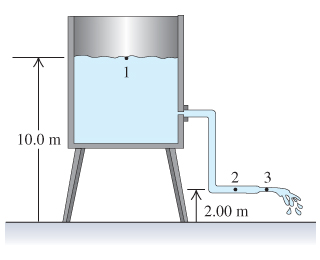# Problem: Water flows steadily from an open tank as shown in the figure. (Figure 1) The elevation of point 1 is 10.0 m, and the elevation of points 2 and 3 is 2.00 m. The cross-sectional area at point 2 is 4.80x10-2 m2; at point 3, where the water is discharged, it is 1.60x10-2 m2. The cross-sectional area of the tank is very large compared with the cross-sectional area of the pipe.What is the gauge pressure pgauge at point 2?

###### FREE Expert Solution

Applying Bernoulli's principle to points 2 and 3:

$\overline{){{\mathbf{P}}}_{{\mathbf{2}}}{\mathbf{+}}\frac{\mathbf{1}}{\mathbf{2}}{\mathbf{\rho }}{{\mathbf{v}}_{\mathbf{2}}}^{{\mathbf{2}}}{\mathbf{+}}{{\mathbf{h}}}_{{\mathbf{2}}}{\mathbf{\rho g}}{\mathbf{=}}{{\mathbf{P}}}_{{\mathbf{0}}}{\mathbf{+}}\frac{\mathbf{1}}{\mathbf{2}}{\mathbf{\rho }}{{\mathbf{v}}_{\mathbf{3}}}^{{\mathbf{2}}}{\mathbf{+}}{{\mathbf{h}}}_{{\mathbf{3}}}{\mathbf{\rho g}}}$

We're looking for P2.

We can solve for P2 as follows:

$\begin{array}{rcl}{\mathbf{P}}_{\mathbf{2}}\mathbf{-}{\mathbf{P}}_{\mathbf{0}}& \mathbf{=}& \frac{\mathbf{1}}{\mathbf{2}}\mathbf{\rho }{{\mathbf{v}}_{\mathbf{3}}}^{\mathbf{2}}\mathbf{-}\frac{\mathbf{1}}{\mathbf{2}}\mathbf{\rho }{{\mathbf{v}}_{\mathbf{2}}}^{\mathbf{2}}\mathbf{+}{\mathbf{\rho gh}}_{\mathbf{3}}\mathbf{-}{\mathbf{\rho gh}}_{\mathbf{2}}\end{array}$

85% (222 ratings)###### Problem Details

Water flows steadily from an open tank as shown in the figure. (Figure 1) The elevation of point 1 is 10.0 m, and the elevation of points 2 and 3 is 2.00 m. The cross-sectional area at point 2 is 4.80x10-2 m2; at point 3, where the water is discharged, it is 1.60x10-2 m2. The cross-sectional area of the tank is very large compared with the cross-sectional area of the pipe.

What is the gauge pressure pgauge at point 2?Frequently Asked Questions

What scientific concept do you need to know in order to solve this problem?

Our tutors have indicated that to solve this problem you will need to apply the Bernoulli's Equation concept. If you need more Bernoulli's Equation practice, you can also practice Bernoulli's Equation practice problems.

What professor is this problem relevant for?

Based on our data, we think this problem is relevant for Professor Stavola's class at LEHIGH.